# Proportions and Similar Figures Section 2 8 Goals

• Slides: 36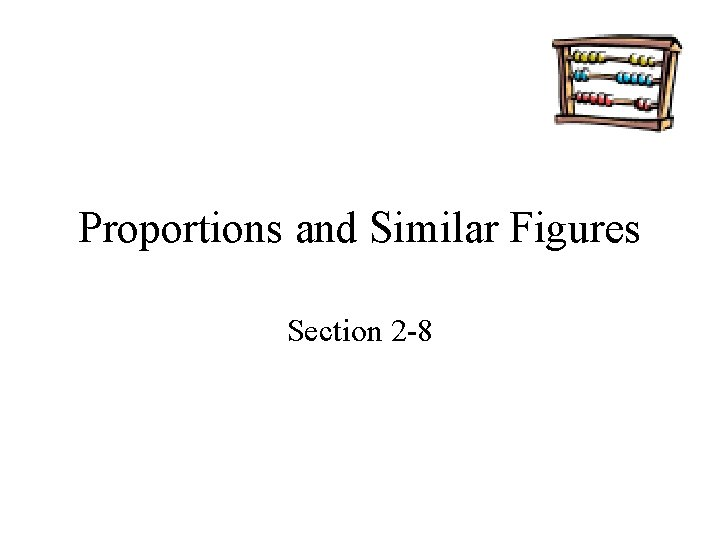Proportions and Similar Figures Section 2 -8Goals Goal Rubric • To find missing lengths in similar figures. • To use similar figures when measuring indirectly. Level 1 – Know the goals. Level 2 – Fully understand the goals. Level 3 – Use the goals to solve simple problems. Level 4 – Use the goals to solve more advanced problems. Level 5 – Adapts and applies the goals to different and more complex problems.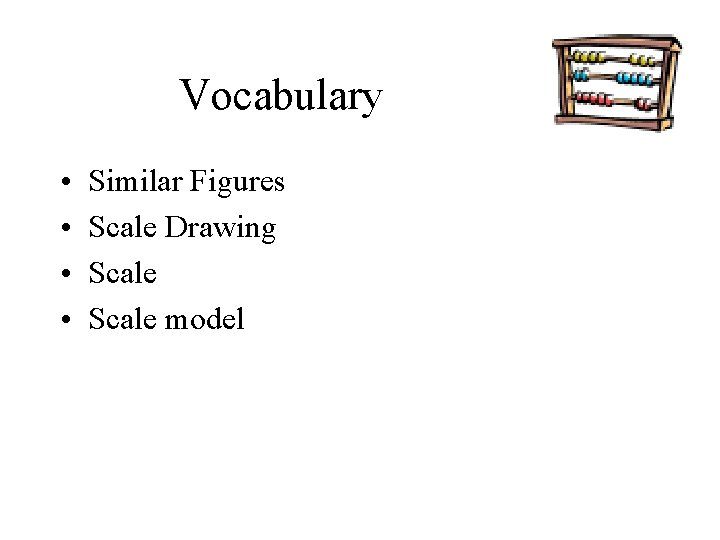Vocabulary • • Similar Figures Scale Drawing Scale model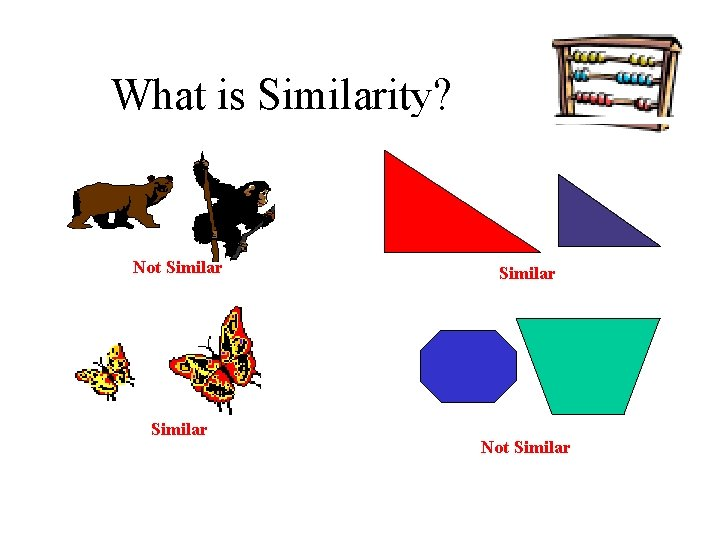What is Similarity? Similar Triangles Not Similar Not SimilarSimilar Figures that have the same shape but not necessarily the same size are similar figures But what does “same shape mean”? Are the two heads similar? N O T Si m ila rSimilar Figures Similar figures can be thought of as enlargements or reductions with no irregular distortions. – So two figures are similar if one can be enlarged or reduced so that it is congruent (means the figures have the same dimensions and shape, symbol ≅) to the original.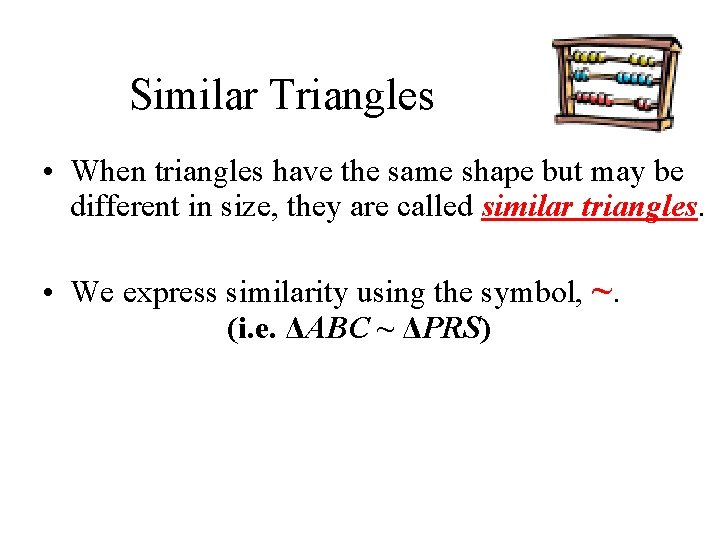Similar Triangles • When triangles have the same shape but may be different in size, they are called similar triangles. • We express similarity using the symbol, ~. (i. e. ΔABC ~ ΔPRS)Example - Similar Triangles Figures that are similar (~) have the same shape but not necessarily the same size.Similar Figures Similar figures have exactly the same shape but not necessarily the same size. Corresponding sides of two figures are in the same relative position, and corresponding angles are in the same relative position. Two figures are similar if and only if the lengths of corresponding sides are proportional and all pairs of corresponding angles have equal measures.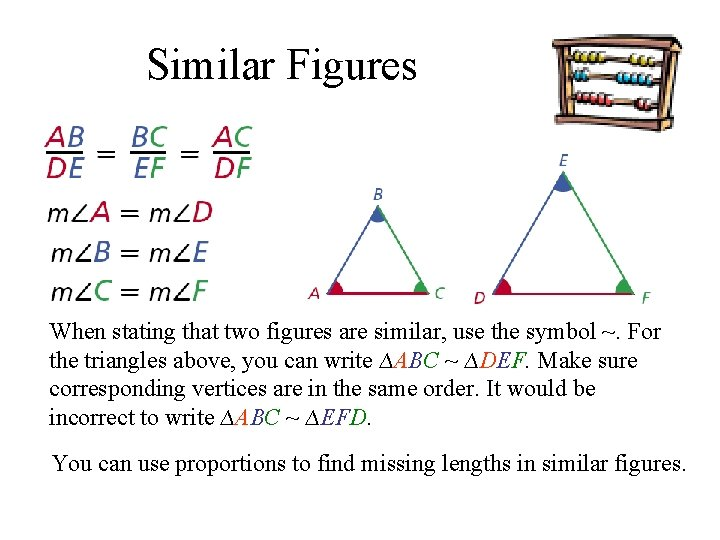Similar Figures When stating that two figures are similar, use the symbol ~. For the triangles above, you can write ∆ABC ~ ∆DEF. Make sure corresponding vertices are in the same order. It would be incorrect to write ∆ABC ~ ∆EFD. You can use proportions to find missing lengths in similar figures.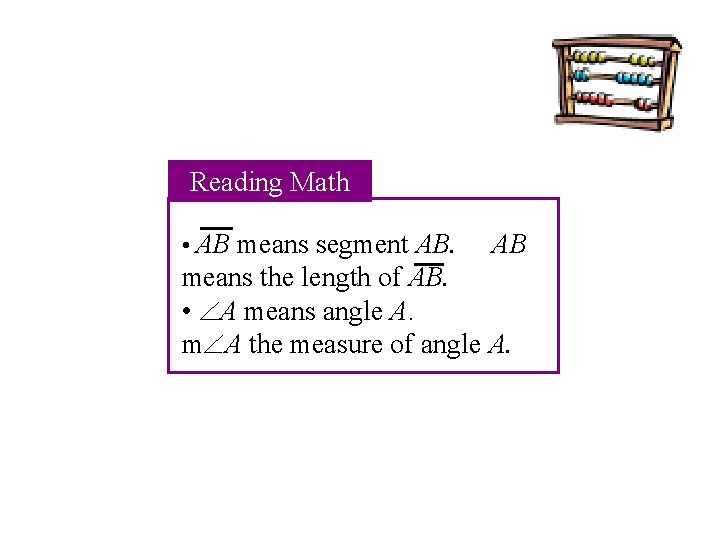Reading Math • AB means segment AB. AB means the length of AB. • A means angle A. m A the measure of angle A.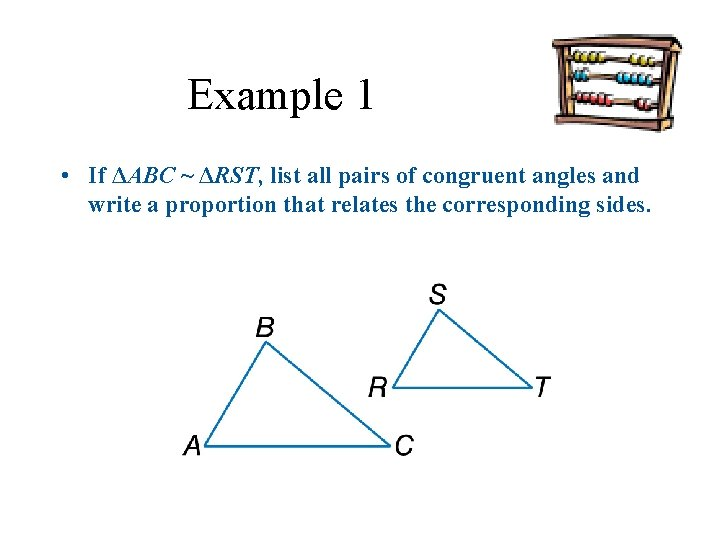Example 1 • If ΔABC ~ ΔRST, list all pairs of congruent angles and write a proportion that relates the corresponding sides.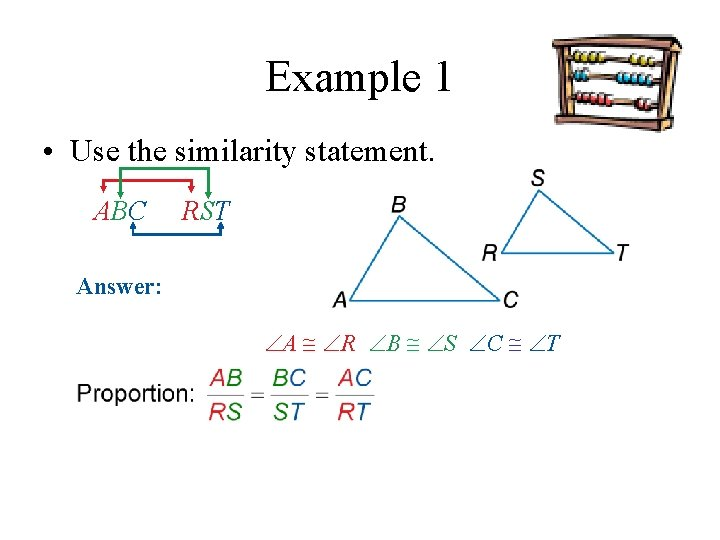Example 1 • Use the similarity statement. ΔABC ~ RST Answer: Congruent Angles: A R, B S, C T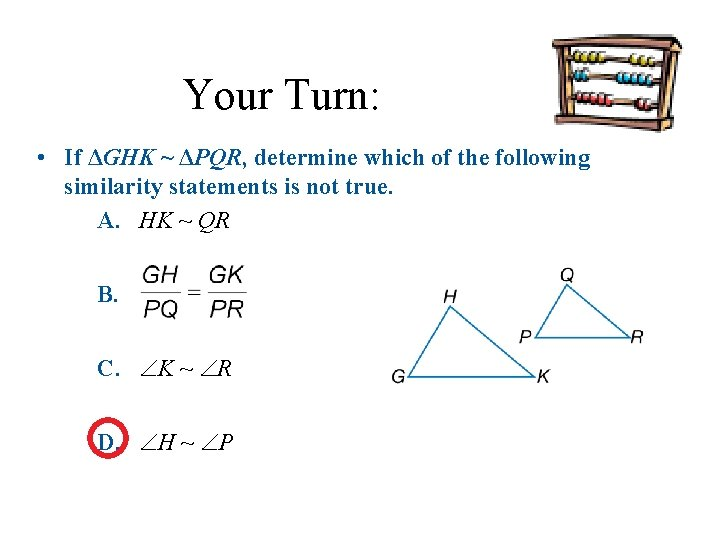Your Turn: • If ΔGHK ~ ΔPQR, determine which of the following similarity statements is not true. A. HK ~ QR B. C. K ~ R D. H ~ P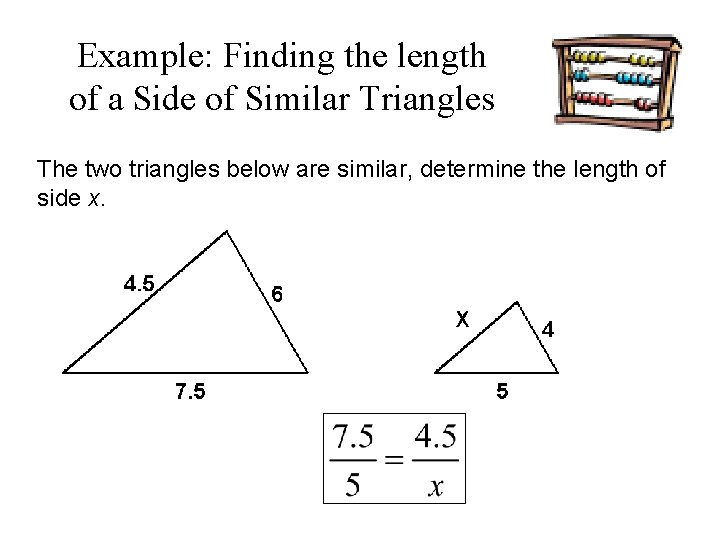Example: Finding the length of a Side of Similar Triangles The two triangles below are similar, determine the length of side x.Example: Continued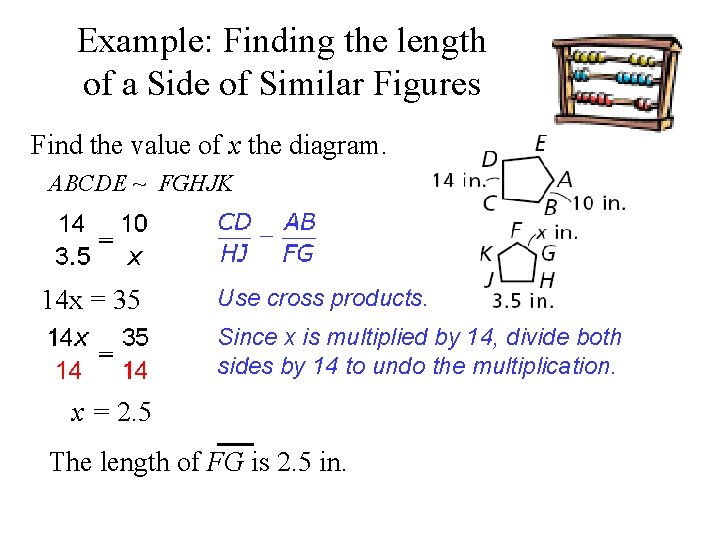Example: Finding the length of a Side of Similar Figures Find the value of x the diagram. ABCDE ~ FGHJK 14 x = 35 Use cross products. Since x is multiplied by 14, divide both sides by 14 to undo the multiplication. x = 2. 5 The length of FG is 2. 5 in.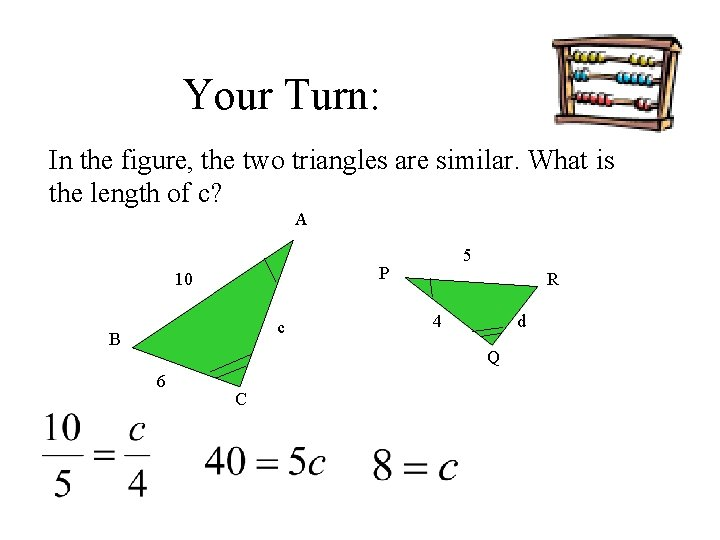Your Turn: In the figure, the two triangles are similar. What is the length of c? A 5 P 10 c B R 4 d Q 6 CYour Turn: In the figure, the two triangles are similar. What is the length of d? A 5 P 10 c B R 4 d Q 6 C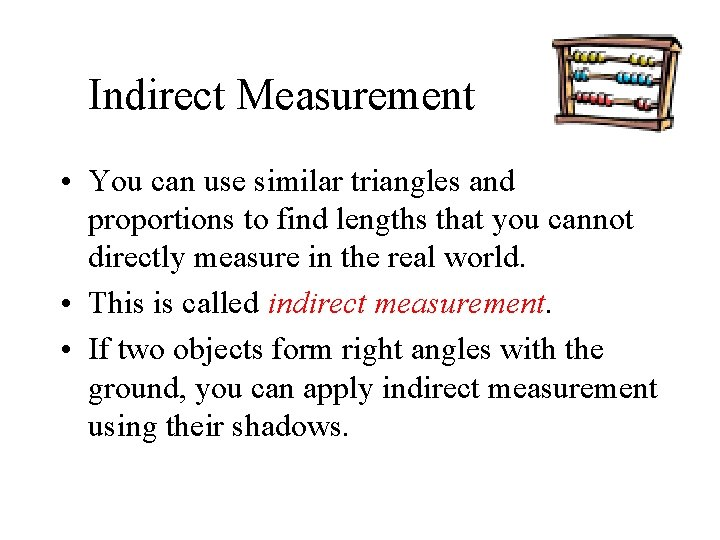Indirect Measurement • You can use similar triangles and proportions to find lengths that you cannot directly measure in the real world. • This is called indirect measurement. • If two objects form right angles with the ground, you can apply indirect measurement using their shadows.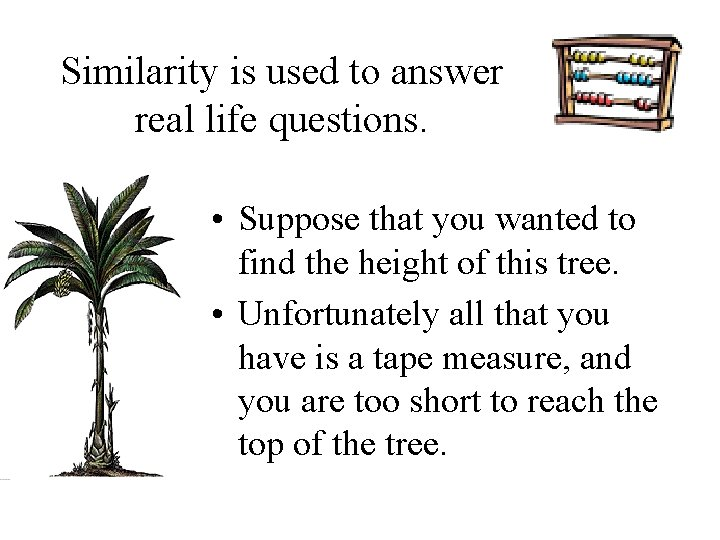Similarity is used to answer real life questions. • Suppose that you wanted to find the height of this tree. • Unfortunately all that you have is a tape measure, and you are too short to reach the top of the tree.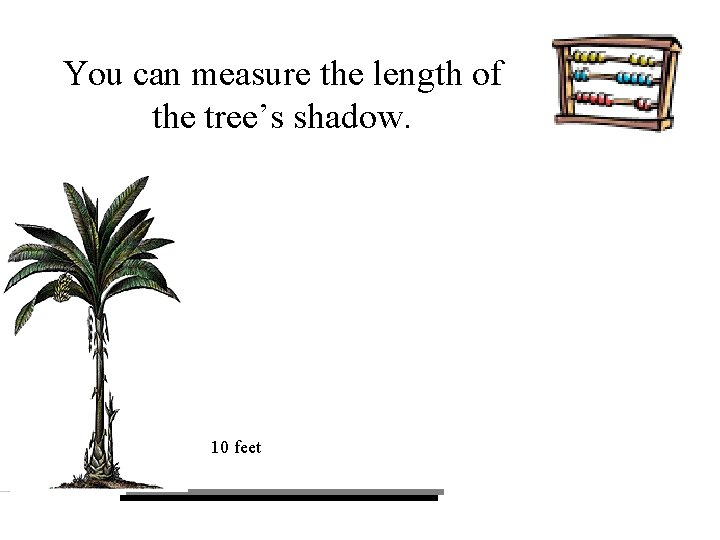You can measure the length of the tree’s shadow. 10 feet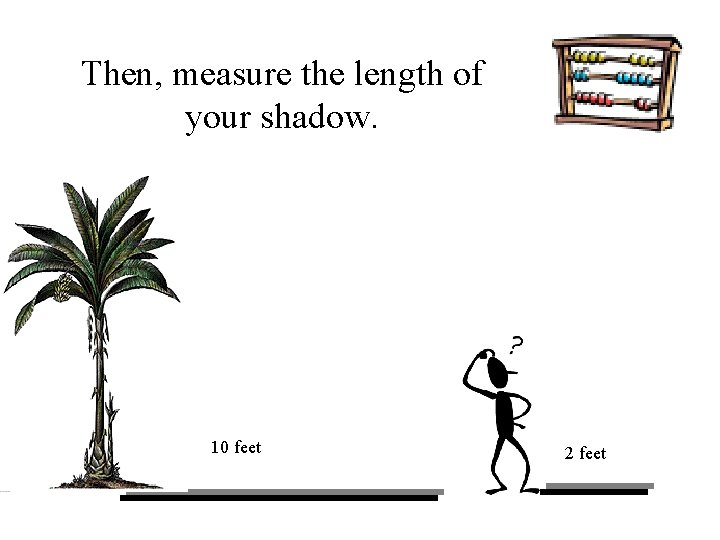Then, measure the length of your shadow. 10 feet 2 feet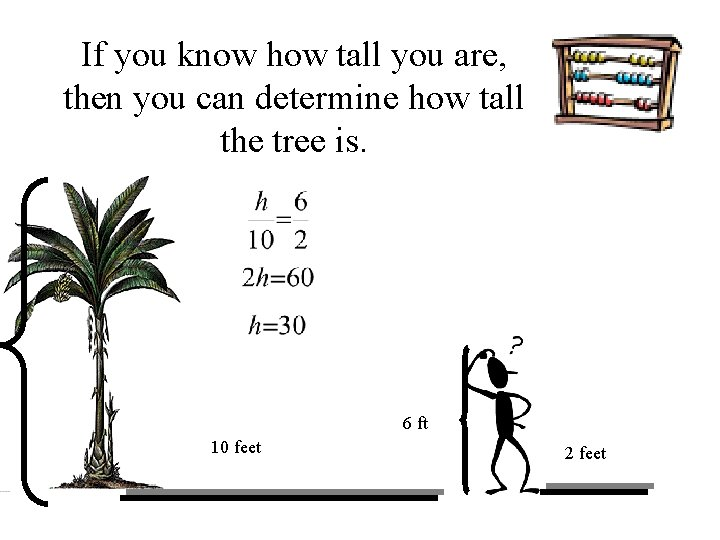If you know how tall you are, then you can determine how tall the tree is. 6 ft 10 feet 2 feet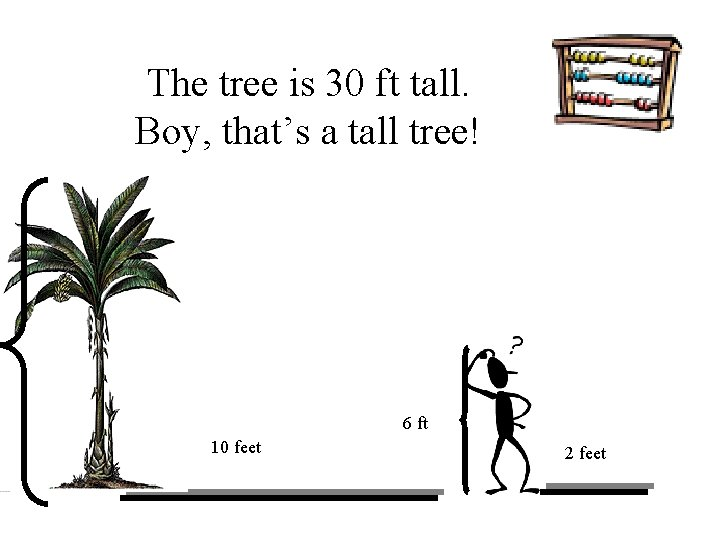The tree is 30 ft tall. Boy, that’s a tall tree! 6 ft 10 feet 2 feet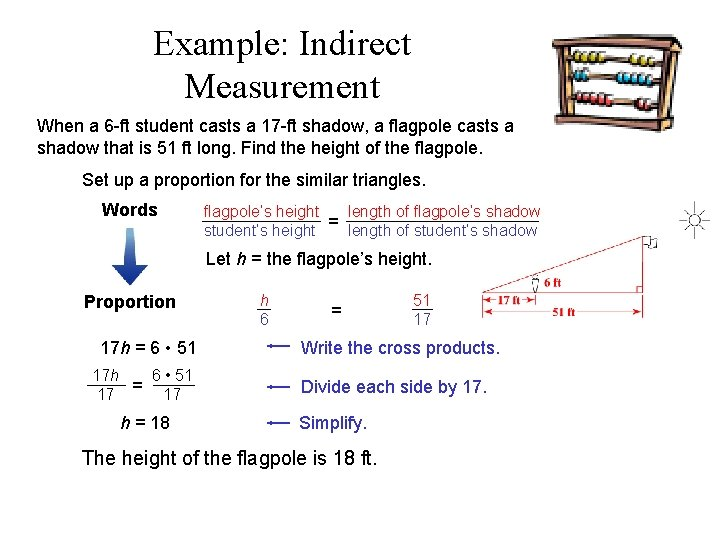Example: Indirect Measurement When a 6 -ft student casts a 17 -ft shadow, a flagpole casts a shadow that is 51 ft long. Find the height of the flagpole. Set up a proportion for the similar triangles. Words flagpole’s height length of flagpole’s shadow = student’s height length of student’s shadow Let h = the flagpole’s height. h 6 Proportion 17 h = 6 • 51 17 h 6 • 51 = 17 17 h = 18 = 51 17 Write the cross products. Divide each side by 17. Simplify. The height of the flagpole is 18 ft.Your Turn: When a 6 -ft student casts a 17 -ft shadow, a tree casts a shadow that is 102 ft long. Find the height of the tree. h 6 17 102Your Turn: 1. Use the similar triangles to find the height of the telephone pole. x 8 ft 6 ft 15 ft 20 feet 2. On a sunny afternoon, a goalpost casts a 75 ft shadow. A 6. 5 ft football player next to the goal post has a shadow 19. 5 ft long. How tall is the goalpost? 25 feet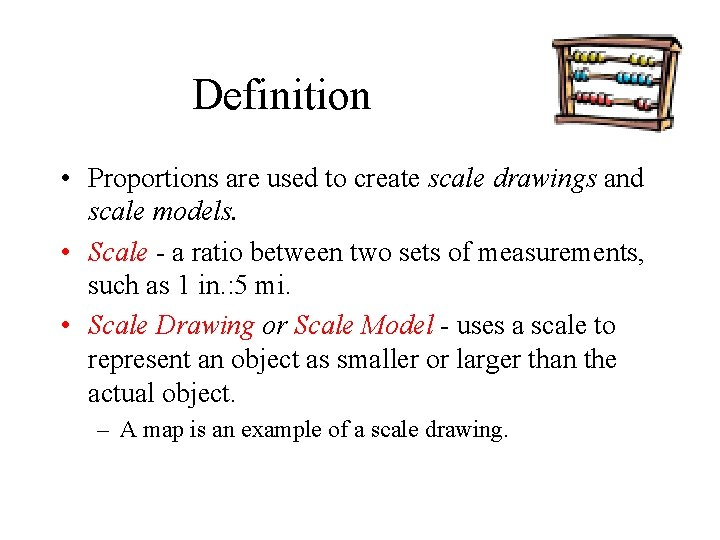Definition • Proportions are used to create scale drawings and scale models. • Scale - a ratio between two sets of measurements, such as 1 in. : 5 mi. • Scale Drawing or Scale Model - uses a scale to represent an object as smaller or larger than the actual object. – A map is an example of a scale drawing.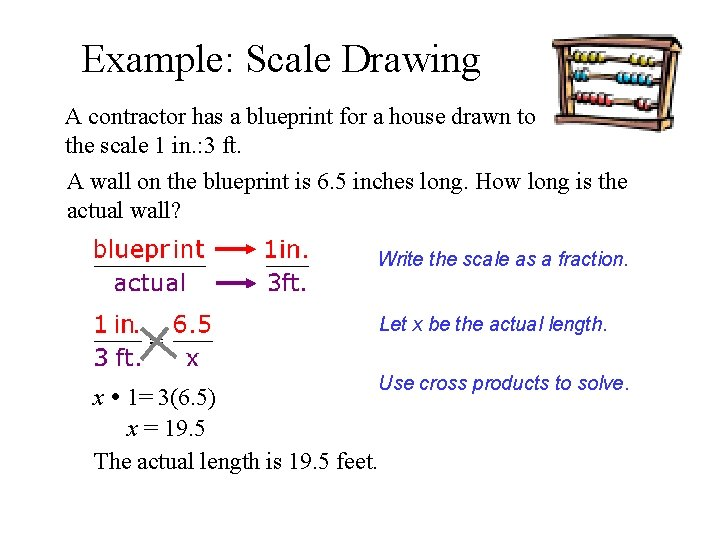Example: Scale Drawing A contractor has a blueprint for a house drawn to the scale 1 in. : 3 ft. A wall on the blueprint is 6. 5 inches long. How long is the actual wall? Write the scale as a fraction. Let x be the actual length. Use cross products to solve. x 1= 3(6. 5) x = 19. 5 The actual length is 19. 5 feet.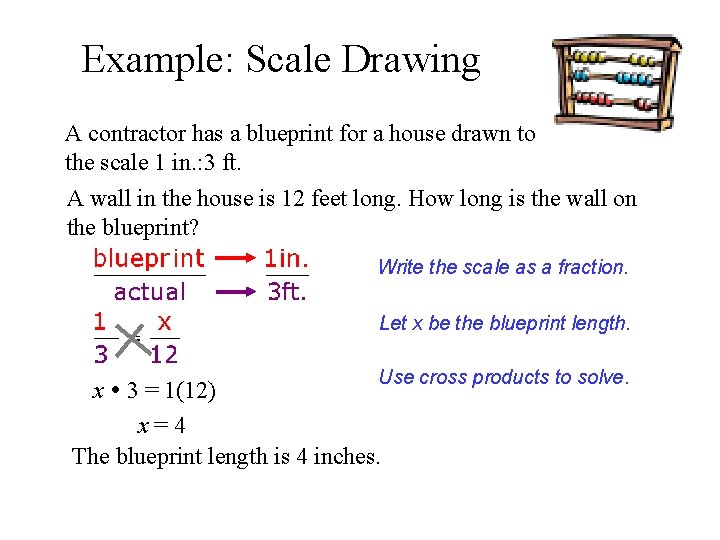Example: Scale Drawing A contractor has a blueprint for a house drawn to the scale 1 in. : 3 ft. A wall in the house is 12 feet long. How long is the wall on the blueprint? Write the scale as a fraction. Let x be the blueprint length. Use cross products to solve. x 3 = 1(12) x=4 The blueprint length is 4 inches.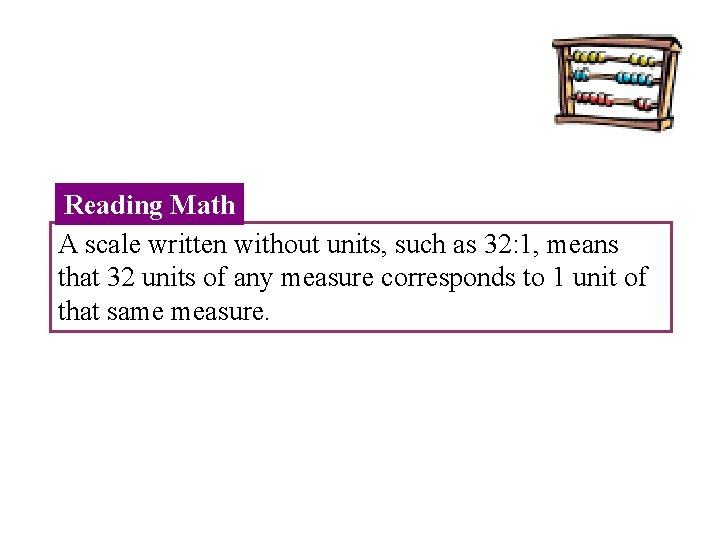Reading Math A scale written without units, such as 32: 1, means that 32 units of any measure corresponds to 1 unit of that same measure.Your Turn: The actual distance between North Chicago and Waukegan is 4 mi. What is the distance between these two locations on the map? Write the scale as a fraction. Let x be the map distance. 18 x = 4 Use cross products to solve. x ≈ 0. 2 The distance on the map is about 0. 2 in.Your Turn: A scale model of a human heart is 16 ft long. The scale is 32: 1 How many inches long is the actual heart that the model represents? Write the scale as a fraction. Let x be the actual distance. 32 x = 16 x = 0. 5 Use cross products to solve. The actual heart is 0. 5 feet or 6 inches.Joke Time • What kind of coffee was served on the Titanic? • Sanka. • And what kind of lettuce was served on the Titanic? • Iceberg. • Why do gorillas have big nostrils? • Because they have big fingers.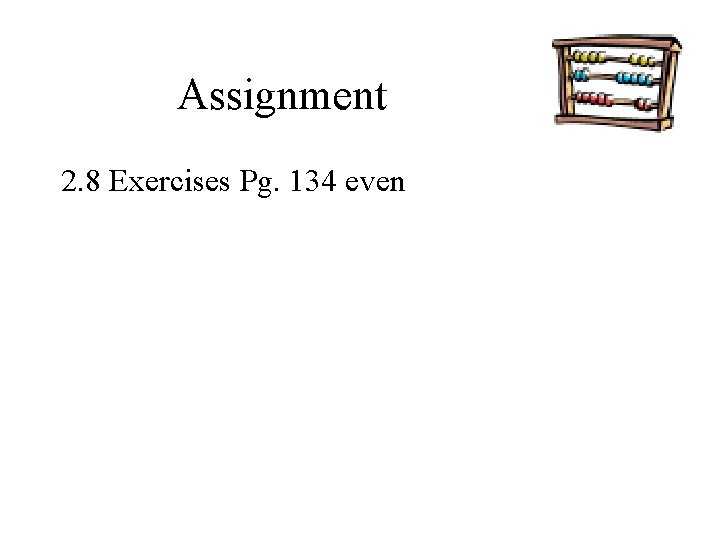Assignment 2. 8 Exercises Pg. 134 even